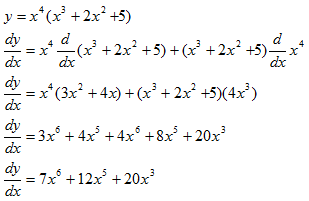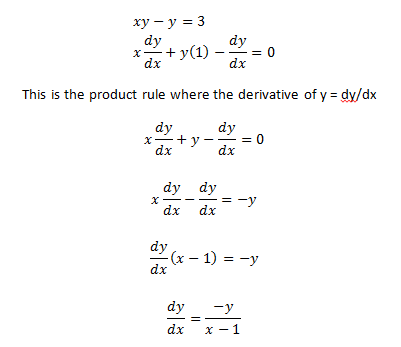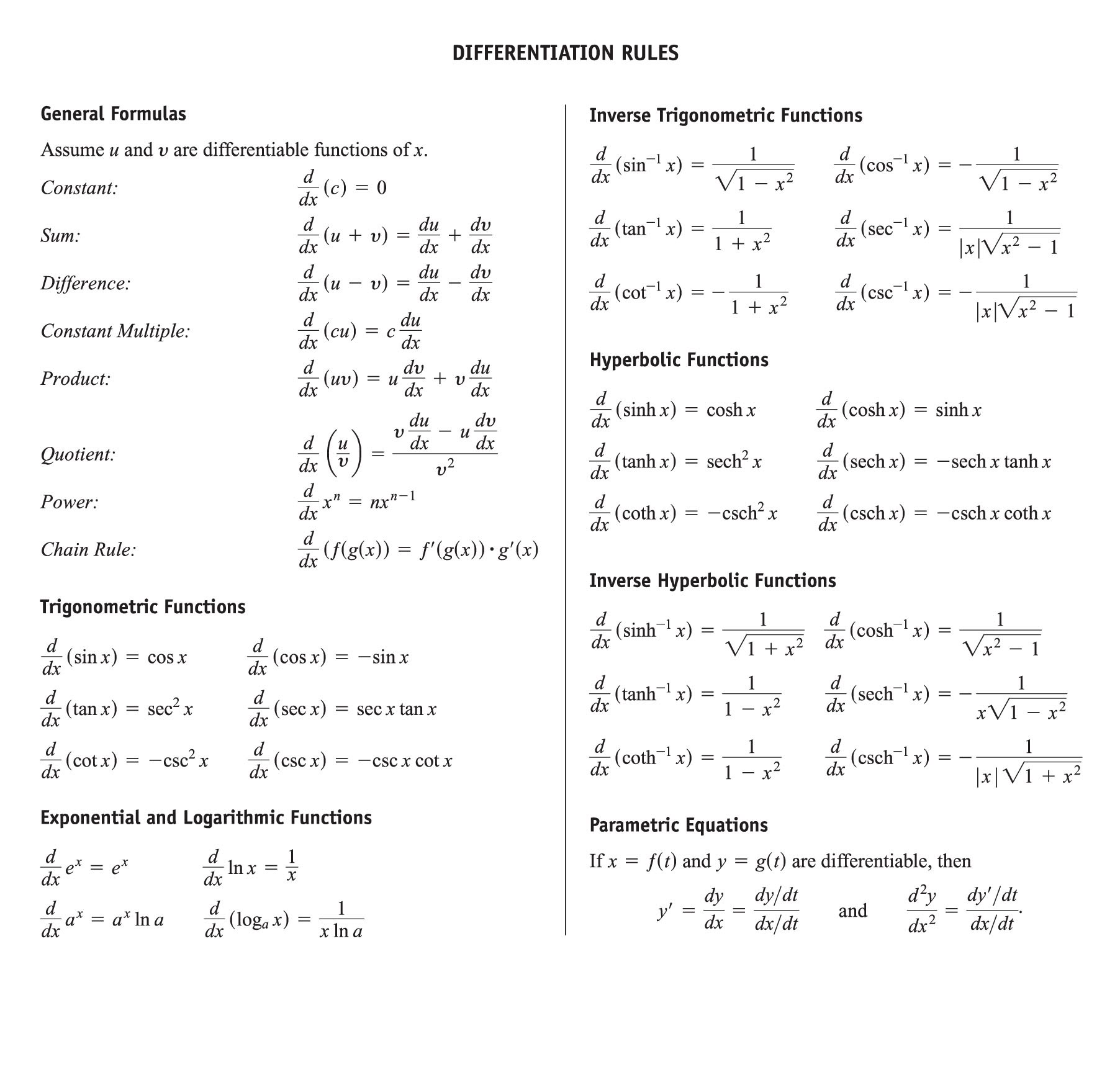### Differentiation

Rate of change velocity with respect to time (which is acceleration) is called Differentiation. For example, it allows us to find the rate of change, to find the rate of change of x with respect to y, which on a graph of y against x is the gradient of the curve. There are a number of simple rules.

If y = some function of x (in other words if y is equal to an expression containing numbers and x’s), then the derivative of y (with respect to x) is written dy/dx, pronounced “dee y by dee x”

Differentiating x to the power of something

1) If y = xn, so that dy/dx = nxn-1

2) If y = kxn, so that, dy/dx = nkxn-1(where k is a constant- in other words a number)

Therefore to differentiate x to the power of something you bring the power down to in front of the x, and then reduce the power by one.

Some examples of differentiation:

1.Find the differentiation of y=x4(x3+2x2+5)

Solution:In this solution product rule of differentiation used

​​ [if y=f(x).g(x) than dy/dx=f(x)[dg(x)/dx]+g(x) [df(x)/dx]]2. Find the differentiation of xy-y=3

Solution: In this solution product rule of differentiation usedSome differentiation Rules: